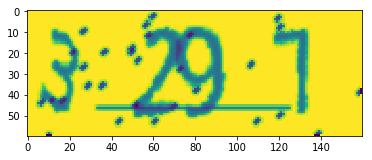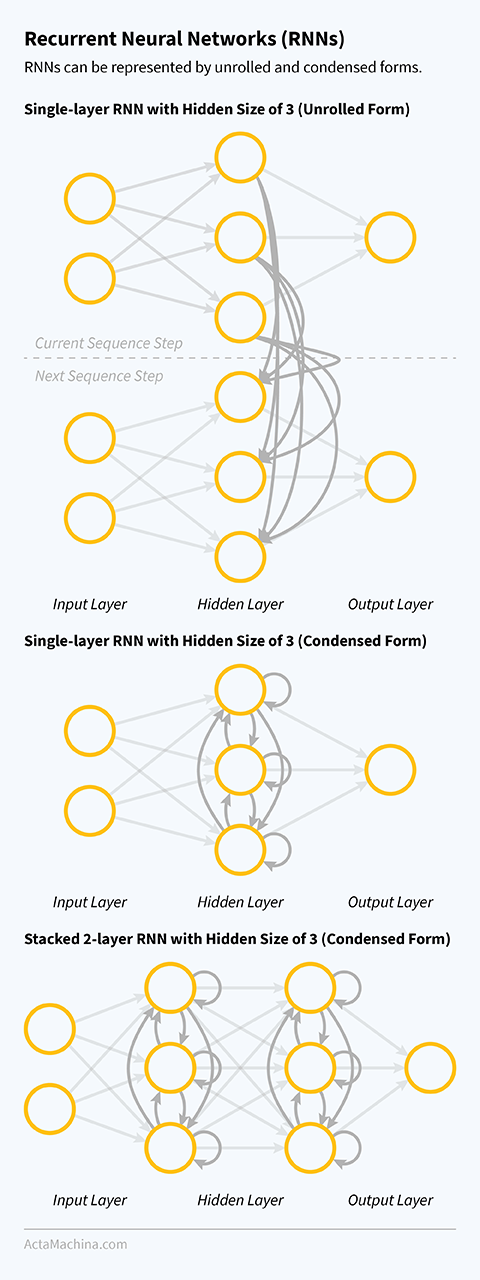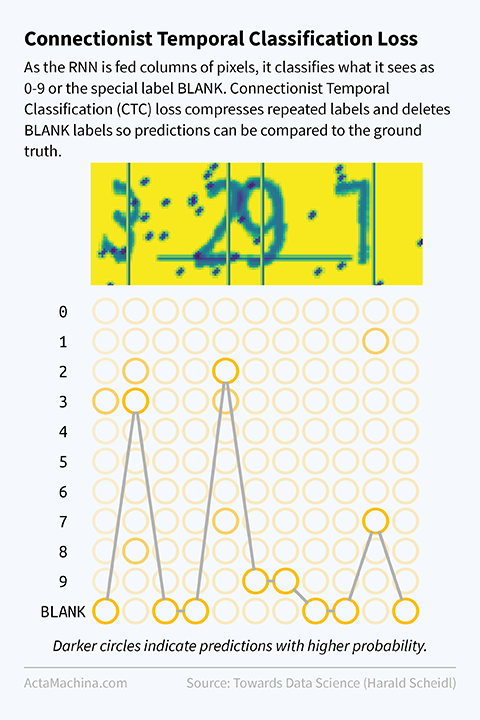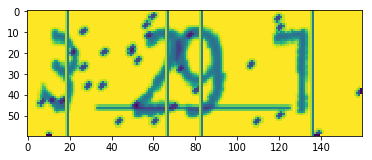This post introduces sequence to sequence prediction. In previous posts, our models have only made single predictions in the form of a class (for classification) or a quantity (for regression). By adopting a Long Short-Term Memory (LSTM) architecture for the Neural Network and Connectionist Temporal Classification (CTC) loss for the loss function, we can read in a sequence and predict a sequence.

More specifically, we'll take a CAPTCHA image as input and predict the numbers in that image as output. CAPTCHAs (short for Completely Automated Public Turing test to tell Computers and Humans Apart) are used by websites to determine whether a user is human or not, most often to prevent spam bots from submitting fake forms online. Google has famously used it's own version of CAPTCHA (called reCATPCHA) to digitise the archives of The New York Times and books from its own Google Books collection.

In this post, you'll learn how to:

• build a Long Short Term Memory (LSTM) neural network;
• train a network using Connectionist Temporal Classification (CTC) loss.

By the end, you'll learn to decode the numbers in:

Out:
<matplotlib.image.AxesImage at 0x7fbaec7c9898>## Import Packages¶

In :
from itertools import groupby

from torchvision import transforms
from torch.utils.data.dataset import random_split

import matplotlib.pyplot as plt

import torch
import torch.nn as nn
import torch.nn.functional as F
import torch.optim as optim


Given a string, the Python package captcha can generate a CAPTCHA image with that string. Here, we generate CAPTCHA images for all 4 digit numbers, saving the number in the file name:

In :
from captcha.image import ImageCaptcha

for chars in range(0, 10000):
image.write(f'{chars:>04}', f'{chars:>04}.png')


Wrapping the CAPTCHA images in a PyTorch dataset:

In :
class CaptchaDataset(Dataset):

def __init__(self, root_dir, transform=None):
self.root_dir = root_dir
self.image_paths = list(Path(root_dir).glob('*'))
self.transform = transform

def __getitem__(self, index):
image = Image.open(self.image_paths[index])

if self.transform:
image = self.transform(image)

label_sequence = [int(c) for c in self.image_paths[index].stem]
return (image, torch.tensor(label_sequence))

def __len__(self):
return len(self.image_paths)


Let's load the dataset and preview the first item:

To be able to normalise the dataset, we need to calculate the mean and variance across the entire dataset:

In :
transform = transforms.Compose([
transforms.Grayscale(),
transforms.ToTensor(),
])

for batch_index, (inputs, labels) in enumerate(dataloader):
print(f'Mean: {inputs.mean()}, Variance: {inputs.std()}')

Mean: 0.8909896016120911, Variance: 0.14787691831588745


Now, let's use the mean, variance and data from CaptchaDataset to create train and test dataloaders:

In :
transform = transforms.Compose([
transforms.Grayscale(),
transforms.ToTensor(),
transforms.Normalize((0.89099,), (0.14788,)),
])

train_dataset, test_dataset = random_split(dataset, [128*64, 28*64])  # total images: 9984



## Long Short Term Memory (LSTM) Neural Networks¶

First, let's set the device:

In :
device = torch.device('cuda:0' if torch.cuda.is_available() else 'cpu')


We'll be making use of a neural network architecture called Stacked Long Short Term Memory (LSTM). But before that, let's start by looking at Recurrent Neural Networks (RNN), which is a general form of the LSTM network.

When humans process sequences of text or images, like reading a sentence or watching a video, they don't examine each word or image individually. Remembering what was processed earlier in a sequence helps us understand things that occur later in the sequence. RNNs are neural networks that contain loops so information from the past affects the current output. One way to visualise a RNN is as multiple copies of the same neural network with fully connected links between them.

Out:The Long Short Term Memory (LSTM) neural networks are a specific form of RNNs. While RNNs can learn from past information if the information was quite recent, it often fails to incorporate information that appears much earlier in the sequence. LSTM networks resolve this issue and allows the network to learn from long-term dependencies. It does this by replacing the single neural network layer found in an unstacked RNN with four neural network layers that interact with each other.

In :
class StackedLSTM(nn.Module):
def __init__(self, input_size=60, output_size=11, hidden_size=512, num_layers=2):
super(StackedLSTM, self).__init__()
self.hidden_size = hidden_size
self.num_layers = num_layers

self.dropout = nn.Dropout()
self.fc = nn.Linear(hidden_size, output_size)
self.lstm = nn.LSTM(input_size, hidden_size, num_layers)

def forward(self, inputs, hidden):
batch_size, seq_len, input_size = inputs.shape
outputs, hidden = self.lstm(inputs, hidden)
outputs = self.dropout(outputs)
outputs = torch.stack([self.fc(outputs[i]) for i in range(width)])
outputs = F.log_softmax(outputs, dim=2)
return outputs, hidden

def init_hidden(self, batch_size):
weight = next(self.parameters()).data
return (weight.new(self.num_layers, batch_size, self.hidden_size).zero_(),
weight.new(self.num_layers, batch_size, self.hidden_size).zero_())

net = StackedLSTM().to(device)


## Connectionist Temporal Classification (CTC) Loss¶

Setting the criterion and optimizer:

In :
criterion = nn.CTCLoss(blank=10)
optimizer = optim.SGD(net.parameters(), lr=0.01, momentum=0.9)


For the criterion, we have replaced our usual nn.CrossEntropyLoss() with nn.CTCLoss(). While Cross Entropy Loss compares a single input with a single output, Connectionist Temporal Classification (CTC) loss lets you compare an input sequence with an output sequence without the need to align them together. Previously, in order to classify sequences like CAPTCHA images, people have used rules to segment the characters with a bounding box first before recognising each digit individually, like in our previous MNIST classifier. Now, using CTC loss, we are able to train the classifer end-to-end, without any immediate steps.

The idea behind CTC loss is to make a classification at each step in the sequence. In our case, each column of pixels in the CAPTCHA image is a step in the sequence and is read from left-to-right. The classifier can choose from the available labels (in our case, 0-9), plus a special BLANK label (denoted by "-") meaning that the classifier couldn't make a prediction.

An example of what the classifier may predict from sequentially classifying a 32 pixel wide CAPTCHA image:

--00000-11---1122222--

The trick to extracting a meaningful prediction from the raw predictions is to collapse repeated characters:

-0-1-12-

And then removing the BLANK labels:

0112

Loss functions require a quantity to optimise. To compute that quantity, we make a list of all valid sequences that match the target. For example, if the target in the above example were indeed 0112, then other valid sequences to reach the same target would include:

--0000--1----1122222-- --0000--1----11---22-- --0000--1-11111---22--

Since the classifier would have a probabilities for each possible label at each step in the sequence, these can be multiplied together to get an overall probability for each of the valid sequences. The quantity for the loss function to optimise then, is the sum of the overall probabilities for all valid sequences. Although the list of valid sequences can be very long, the calculation can be improved using a dynamic programming algorithm.

Out:Unfortunately, PyTorch requires the BLANK label to be an integer, so instead of "-", we assign it to be 10:

In :
BLANK_LABEL = 10


## Training and Testing the Model¶

### Training Phase¶

In [ ]:
net.train()  # set network to training phase

epochs = 30
batch_size = 64

# for each pass of the training dataset
for epoch in range(epochs):
train_loss, train_correct, train_total = 0, 0, 0

h = net.init_hidden(batch_size)

# for each batch of training examples
for batch_index, (inputs, targets) in enumerate(train_dataloader):
inputs, labels = inputs.to(device), labels.to(device)
h = tuple([each.data for each in h])

batch_size, channels, height, width = inputs.shape

# reshape inputs: NxCxHxW -> WxNx(HxC)
inputs = (inputs
.permute(3, 0, 2, 1)
.contiguous()
.view((width, batch_size, -1)))

outputs, h = net(inputs, h)  # forward pass

# compare output with ground truth
input_lengths = torch.IntTensor(batch_size).fill_(width)
target_lengths = torch.IntTensor([len(t) for t in targets])
loss = criterion(outputs, targets, input_lengths, target_lengths)

loss.backward()  # backpropagation
optimizer.step()  # update network weights

# record statistics
prob, max_index = torch.max(outputs, dim=2)
train_loss += loss.item()
train_total += len(targets)

for i in range(batch_size):
raw_pred = list(max_index[:, i].cpu().numpy())
pred = [c for c, _ in groupby(raw_pred) if c != BLANK_LABEL]
target = list(targets[i].cpu().numpy())
if pred == target:
train_correct += 1

# print statistics every 10 batches
if (batch_index + 1) % 10 == 0:
print(f'Epoch {epoch + 1}/{epochs}, ' +
f'Batch {batch_index + 1}/{len(train_dataloader)}, ' +
f'Train Loss: {(train_loss/1):.5f}, ' +
f'Train Accuracy: {(train_correct/train_total):.5f}')

train_loss, train_correct, train_total = 0, 0, 0


### Testing Phase¶

In [ ]:
h = net.init_hidden(batch_size)  # init hidden state

net.eval()

test_loss = 0
test_correct = 0

# for each batch of testing examples
for batch_index, (inputs, targets) in enumerate(test_dataloader):
inputs, labels = inputs.to(device), labels.to(device)
h = tuple([each.data for each in h])
batch_size, channels, height, width = inputs.shape

# reshape inputs: NxCxHxW -> WxNx(HxC)
inputs = (inputs
.permute(3, 0, 2, 1)
.contiguous()
.view((width, batch_size, -1)))

outputs, h = net(inputs, h)  # forward pass

# record loss
input_lengths = torch.IntTensor(batch_size).fill_(width)
target_lengths = torch.IntTensor([len(t) for t in targets])
loss = criterion(outputs, targets, input_lengths, target_lengths)
test_loss += loss.item()

# compare prediction with ground truth
prob, max_index = torch.max(outputs, dim=2)

for i in range(batch_size):
raw_pred = list(max_index[:, i].cpu().numpy())
pred = [c for c, _ in groupby(raw_pred) if c != BLANK_LABEL]
target = list(targets[i].cpu().numpy())
if pred == target:
test_correct += 1

f'Test Accuracy: {(test_correct/test_total):.5f} ' +
f'({test_correct}/{test_total})')


Now, let's load the CAPTCHA image mentioned at the start and the ground truth target:

In :
data_iterator = iter(test_dataloader)
inputs, targets = data_iterator.next()

i = 1

image = inputs[i,0,:,:]

print(f"Target: {''.join(map(str, targets[i].numpy()))}")
plt.imshow(image)

Target: 3291

Out:
<matplotlib.image.AxesImage at 0x7fae5d569cf8>And let's make a prediction using our trained LSTM neural network:

In :
inputs = inputs.to(device)

batch_size, channels, height, width = inputs.shape
h = net.init_hidden(batch_size)

inputs = (inputs
.permute(3, 0, 2, 1)
.contiguous()
.view((width, batch_size, -1)))

# get prediction
outputs, h = net(inputs, h)  # forward pass
prob, max_index = torch.max(outputs, dim=2)
raw_pred = list(max_index[:, i].cpu().numpy())

# print raw prediction with BLANK_LABEL replaced with "-"
print('Raw Prediction: ' + ''.join([str(c) if c != BLANK_LABEL else '-' for c in raw_pred]))

pred = [str(c) for c, _ in groupby(raw_pred) if c != BLANK_LABEL]
print(f"Prediction: {''.join(pred)}")


Raw Prediction: -------------------33----------------------------------------------222-------------999--------------------------------------------------77----------------------
Prediction: 3297


Out of interest, let's annotate the CAPTCHA image with lines where the digits are first recognised:

In :
line_mask = [(a == BLANK_LABEL) & (b != BLANK_LABEL) for a, b in zip(raw_pred, raw_pred[1:])]
indices = [i for i, x in enumerate(line_mask) if x]

annotated_image = image.clone()
for index in indices:
annotated_image[:, index] = 0
annotated_image[:, index+1] = -2

plt.imshow(annotated_image)

Out:
<matplotlib.image.AxesImage at 0x7fbadc7d3a90>## Summary and Next Steps¶

In this post, you've learnt how to: